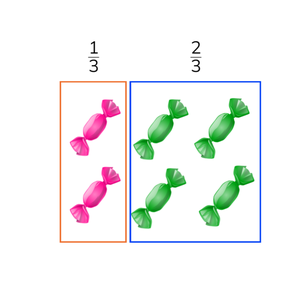Determine a total with simple fractions

# Determine a total with simple fractions8,000 schools use Gynzy92,000 teachers use Gynzy1,600,000 students use Gynzy

## General

Students learn to determine the total using a simple fraction.

## Common core standard(s)

CCSS.MATH.CONTENT.4.NF.A.1,
CCSS.MATH.CONTENT.5.NF.B.4

## Introduction

Students determine the fractions shown by the fraction bar.

## Development

Refresh the composition of a fraction with the class. Fractions are composed of a numerator on top and a denominator on the bottom. (Tip- tell students to think of "Down-ominator to remember that the denominator is on the bottom.) Explain to students that if you want to determine the total using a simple fraction that the first step is to determine how much 1 segment of the fraction is. In the example of 2//5 you first calculate what 1//5 is. Is you know that 2//5 marbles is 4 marbles, then you know that 1//5 is 2 marbles. You divide both by 2. Now you can calculate how much the total (5//5) is. You multiply by 5. You also multiply the amount of marbles by 5. So 2 x 5 = 10 marbles. Calculate the next exercise with the students. You can do this step by step, by removing the covers on the interactive whiteboard.Check that students are able to calculate this by asking them to solve the next problem. Also ask students to explain their strategy as they work. Next explain how to solve this kind of fraction problem when in story form. Explain that you first determine how many objects belong to each segment of the fraction. In this example 3//4 equals 6 dogs. Show that you calculate what 1//4 is by dividing by 3. You also do this with the total. So 6 ÷ 3 = 2. 1//4 equals 2 dogs. You then multiply by 4 to find the total (8 dogs). Ask students to solve the next two problems.

Check that students understand how to determine the total from a simple fraction by asking the following question:
- Which steps do you use to determine the total when given a simple fraction?

## Guided practice

Students practice exercises with a fraction bar, then with abstract fractions with a visual and then are given fraction story problems.

## Closing

Discuss with students the importance of being able to calculate a total from a given simple fraction. To close ask students to work in groups to determine who of the students presented on the interactive whiteboard had the largest bar of chocolate with the most chocolate squares.

## Teaching tips

Students who have difficulty determining the total from the simple fraction can first practice with determining what the simple fraction is. They can use fraction circles or bars to help visualize the fractions.

### The online teaching platform for interactive whiteboards and displays in schools

• Save time building lessons

• Manage the classroom more efficiently

• Increase student engagement